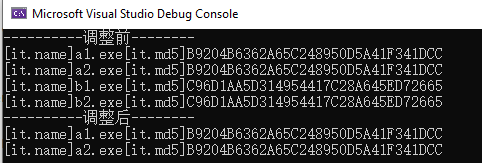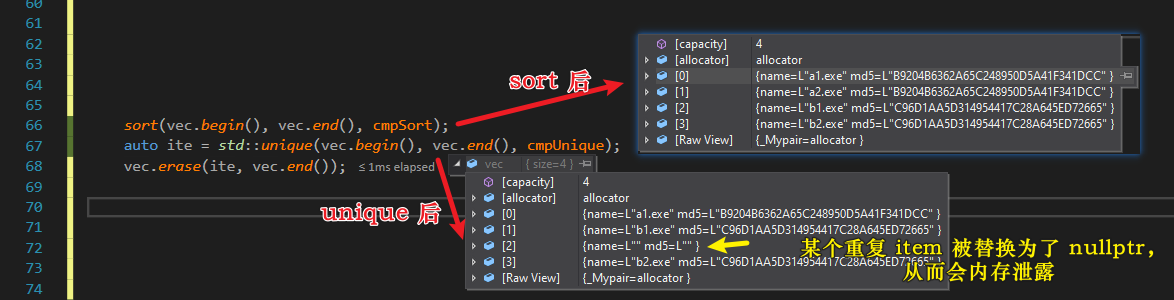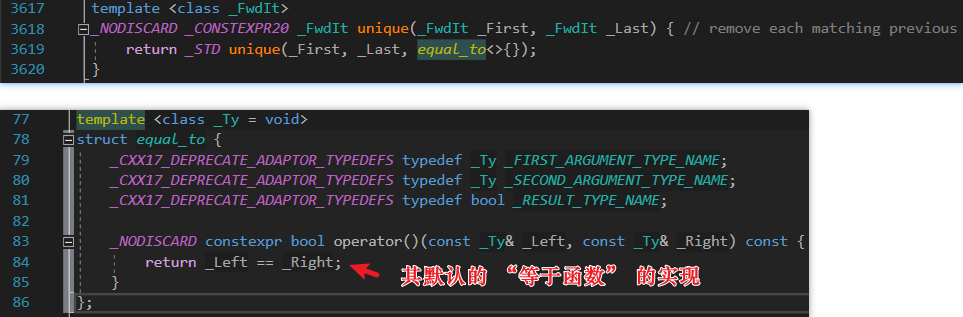1. C++ `std::unique` 函数去重，却导致的 `std::vector` 发生改变（遇内存泄漏）
2. c++ `std::vector` 利用 `std::set` 去重（自定义结构体创建 set 对象的方法）
3. 自定义结构体在 `sort``unique``比较 / 等于` 函数书写（重载、函数、函数对象；严格弱序、相等）

[TOC]

### 背景

在开发防病毒业务中，需要对传入到 `vector` 中的样本进行去重 ；本篇全程采用 C++ STL 库，未调用 Qt 接口。业务抽象出一个具体例子如下。

### 去重思路

搜索发现，对 `std::vector` 共分为两种，一种基础数据类型，另一种自定义结构体类型。

1. 基础数据类型

SO 提供三种解决方案，推荐使用第二种。见 What’s the most efficient way to erase duplicates and sort a vector?

2. 自定义结构体类型

实际项目中，这种我们更常遇到，才是所需。余下全文重点讲解此。

### 解决方案

1. 在自定义结构体中 重载 `<` 函数，简单，参见代码方式一。略
2. 定义一个比较函数，最为常用；亦有用 Lambda 定义；
3. 使用 函数对象，亦简单，属于 2 的变种，在自定义的函数外面套了一层 `class` 或者 `struct` 罢了。

PS: 因实际项目中的结构体，没有和无法重载 `operator<`， 故 1 不可用。然后决定采用 2 方式。

### 『一』vector, sort + unique

``````// 核心代码
sort(vec.begin(), vec.end(), cmpSort);
auto ite = std::unique(vec.begin(), vec.end(), cmpUnique);
vec.erase(ite, vec.end());``````

``````#include <iostream>
#include <string>
#include <algorithm>
#include <vector>
#include <set>
using namespace std;

struct MyData {
wstring name;
wstring md5;
};

bool cmpSort(const MyData& d1, const MyData& d2) {
return d1.md5 < d2.md5;
};

bool cmpUnique(const MyData& d1, const MyData& d2) {
return d1.md5 == d2.md5;
};

int main()
{
vector<MyData> vec = { { L"a1.exe", L"B9204B6362A65C248950D5A41F341DCC"},
{ L"a2.exe", L"B9204B6362A65C248950D5A41F341DCC"},
{ L"b1.exe", L"C96D1AA5D314954417C28A645ED72665"},
{ L"b2.exe", L"C96D1AA5D314954417C28A645ED72665"} };

wcout.imbue(locale("", LC_CTYPE));
wcout << L"----------调整前--------" << endl;
for (const auto& it : vec)
wcout << L"[it.name]" << it.name << L"[it.md5]" << it.md5 << endl;

sort(vec.begin(), vec.end(), cmpSort);
auto ite = std::unique(vec.begin(), vec.end(), cmpUnique);
vec.erase(ite, vec.end());

wcout << L"----------调整后--------" << endl;
for (const auto& it : vec)
wcout << L"[it.name]" << it.name << L"[it.md5]" << it.md5 << endl;
}````````````bool cmpUnique(const MyData& d1, const MyData& d2) {
//return d1.md5 == d2.md5;                          // 此『不严格相等』会内存泄漏
return d1.md5 == d2.md5 && d1.name == d2.name;      // 此『严格相等』满足语法，却不满足实际业务需求
};``````

本想后面有时间再继续研究下方式一，看看自定义 `cmpUnique（）` 修改达到严格弱序的要求，能够不会有内存泄漏，亦能够完美符合要求。但心里痒痒，总是还有一点困惑，两天后，半夜睡不着，又起来研究了下。

翻了下 `std::unique()` 的默认第三个参数的默认实现，如图，发现就是直接 `==` 比较。那就是严格的对象相等，满足语法和编译，但不满足业务的需要，故这个点也没有疑问了。### 『二』vector + set（手动赋值）

#### cmpSort() 定义在 Class 外

``````// 核心代码
set<MyData, decltype(cmpSort)*>  s(&cmpSort);   // 此种方式比较少见，且第二个 & 若想省略亦可，则编译器亦会自动添加
for (unsigned i = 0; i < vec.size(); ++i)
s.insert(vec[i]);
vec.assign(s.begin(), s.end());``````

#### cmpSort() 定义为 Class 成员变量

``````// 核心代码
set<MyData, decltype(DeDuplication::cmpSort)*>  s(&DeDuplication::cmpSort);  // 本行定义为重点
for (unsigned i = 0; i < m_vec.size(); ++i)
s.insert(m_vec[i]);

m_vec.assign(s.begin(), s.end());``````

``````#include <iostream>
#include <string>
#include <algorithm>
#include <vector>
#include <set>
using namespace std;

struct MyData
{
wstring name;
wstring md5;
};

class DeDuplication
{
private:
static bool cmpSort(const MyData& d1, const MyData& d2) {  // ***** 注意，必须是静态 *****
// Simple example
return d1.md5 < d2.md5;

// Complex sample
//if (d1.md5 != d2.md5)
//    return d1.md5 < d2.md5;
//else
//    return d1.name < d2.name;
};

public:
void finally()
{
wcout.imbue(locale("", LC_CTYPE));
wcout << L"----------调整前--------" << endl;
for (const auto& it : m_vec)
wcout << L"[it.name]" << it.name << L"[it.md5]" << it.md5 << endl;

set<MyData, decltype(DeDuplication::cmpSort)*>  s(&DeDuplication::cmpSort);
for (unsigned i = 0; i < m_vec.size(); ++i)
s.insert(m_vec[i]);

m_vec.assign(s.begin(), s.end());

wcout << L"----------调整后--------" << endl;
for (const auto& it : m_vec)
wcout << L"[it.name]" << it.name << L"[it.md5]" << it.md5 << endl;
};

private:
vector<MyData>  m_vec = { { L"a1.exe", L"B9204B6362A65C248950D5A41F341DCC"},
{ L"a2.exe", L"B9204B6362A65C248950D5A41F341DCC"},
{ L"b1.exe", L"C96D1AA5D314954417C28A645ED72665"},
{ L"b2.exe", L"C96D1AA5D314954417C28A645ED72665"} };
};``````

``Error (active)	E0289	no instance of constructor "std::set<_Kty, _Pr, _Alloc>::set [with _Kty=MyData, _Pr=bool (DeDuplication::**)(const MyData &d1, const MyData &d2), _Alloc=std::allocator<MyData>]" matches the argument list	DeDuplication``

### 『三』vector + set（构造函数）

其性能不如『方式二』，略，可参见 Ref 种的第三种。

### 总结

最后结合实际，采用方式二的　`class` 内定义 `static` 此方式最合宜解决此问题。且附上完整源码。

1. 方式一和方式二的类外定义，见完整源码的项目 Unique

2. 方式二的类内定义，见完整源码的项目 DeDuplication

3. 对于给 `std::set` 创建自定义结构体对象，可见 STL 的 std::set 创建自定义结构体的对象，定义严格弱序的比较函数

### 系列地址

QtExamples 【DeDuplication】

### Ref

: C++ set自定义排序